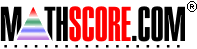Math Practice Online > free > lessons > Florida > 6th grade > Decimals To Fractions

## Decimals To Fractions

This topic deals with being able to convert decimals into simplified fractions. You may need pencil and paper.

 Sample Problems for Decimals To Fractions Lesson for Decimals To Fractions

### This topic aligns to the following state standards

Grade 5: Num 1. Knows that numbers in different forms are equivalent or nonequivalent, using whole numbers, decimals, fractions, mixed numbers, and percents.
Grade 6: Num 4. Converts a number expressed in one form to its equivalent in another form.
Grade 7: Num 4. Converts a number expressed in one form to its equivalent in another form.
Grade 9: Num 4. understands that numbers can be represented in a variety of equivalent forms, including integers, fractions, decimals, percents, scientific notation, exponents, radicals, absolute value, and logarithms

Copyright Accurate Learning Systems Corporation 2008.